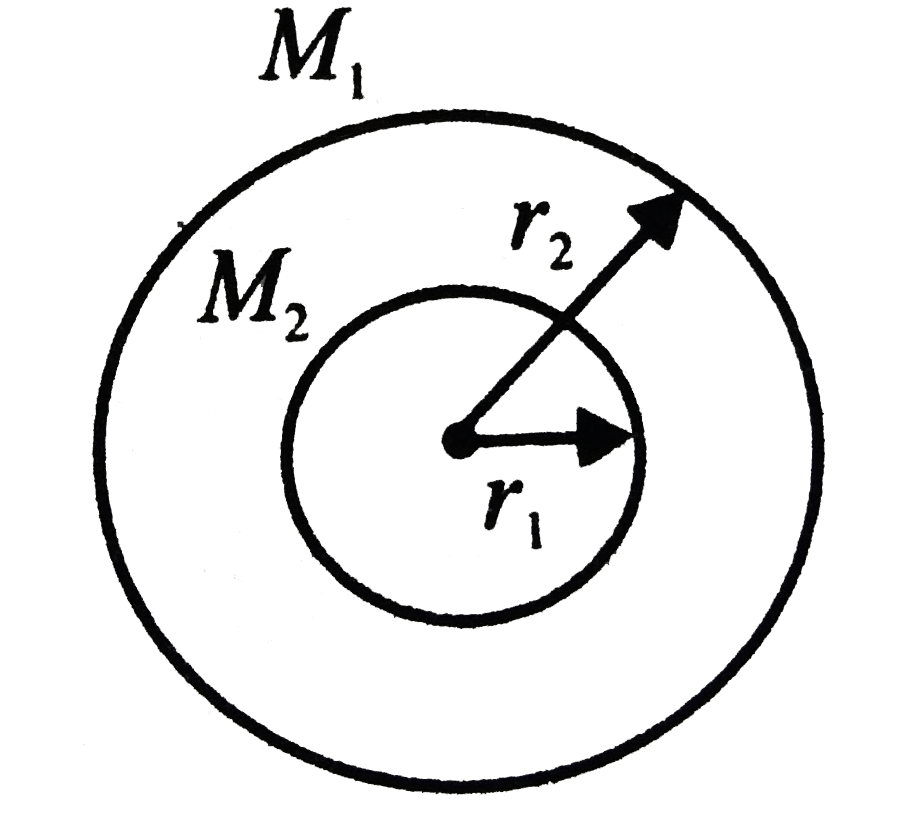# Two concentric shells of masses M_(1) and M_(2) are having radii r_(1) and r_(2). Which of the following is the correct expression for the gra

225 views
in Physics
closed
Two concentric shells of masses M_(1) and M_(2) are having radii r_(1) and r_(2). Which of the following is the correct expression for the gravitational field on a mass m?A. F=(G(M_(1)+M_(2)))/(r^(2)), for rltr_(1)
B. F=(G(M_(1)+M_(2)))/((r^(2)), for rltr_(2)
C. F=(GM_(2))/(r^(2)), for r_(1)ltrltr_(2)
D. F=GM_(1)/(r^(2)), for r_(1)ltrltr_(2)

by (75.0k points)
selected

Gravitational field inside a shell is zero, but for points outside it, the shell behaves as if whole of its mass is concentrated at its centre. Hence, for a point lying in between the shells there will be a field only due to the inner shell (=GM_(2)//r^(2)) because the point will be an external point for the inner shell but internal for the outer shell.